##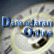Dividend Regressions: January 2017

Variables used in the regression

1. Dividend Yield = Dividends per share in most recent year/ Current Stock Price
2. Dividend Payout Ratio = Dividends / Net Income
3. Beta: Regression or Bottom up beta
4. Expected Growth in EPS over next 5 years = Consensus analyst estimate (or your own) of expected growth in EPS . If you don't have an analyst estimate, use your own estimate of expected growth.
5. Market Debt to Capital = Debt/ (Debt + Market Value of Equity): If you have market value for debt, use it. If not, use book value of debt and market value of equity.

US Regression: Dividend Yield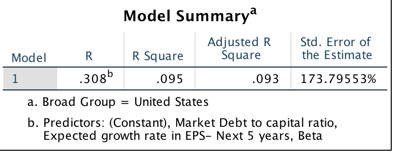### Regression Output

Dividend Yield = .03129 -.01134 Beta - 0.009 Expected Growth +.017 Market Debt to Capital Ratio

T statistic on intercept = 24.27

T statistic on beta = 9.35

T statistic on expected growth = 2.02

T statistic on debt to capital ratio = 7.00

US Regression: Dividend Payout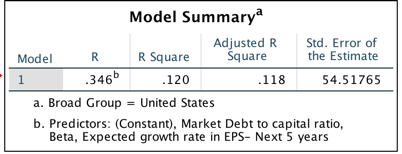### Regression Output

Dividend Yield = .862 -.332 Beta -1.152 Expected Growth +.20 Market Debt to Capital Ratio

T statistic on intercept = 21.40

T statistic on beta = 8.68

T statistic on expected growth = 8.78

T statistic on debt to capital ratio = 2.67

Global Regression: Dividend Yield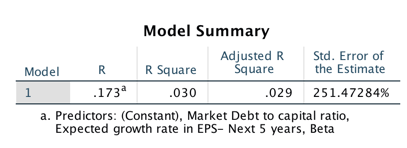### Regression Output

Dividend Yield = .02296 -.0007 Beta - 0.0074 Expected Growth +.019 Market Debt to Capital Ratio

T statistic on intercept = 25.74

T statistic on beta = 1.21

T statistic on expected growth = 3.35

T statistic on debt to capital ratio = 10.62

Global Regression: Dividend Payout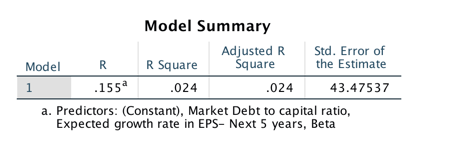### Regression Output

Dividend Yield = .564 -.049 Beta -0.409 Expected Growth +.10 Market Debt to Capital Ratio

T statistic on intercept = 36.08

T statistic on beta = 4.67

T statistic on expected growth = 9.55

T statistic on debt to capital ratio = 4.22

• How do I use this regression?

Assume that you want to estimate the dividend payout ratio for a firm with the following characteristics, using the US regression:

Regression beta = 1.20

Expected Growth in EPS over next 5 years = 10%

Market Debt to Capital = 30%

• Predicted Values
• Expected Dividend Yield = 0.03129 - 0.01134 (1.2) - 0.009 (.10) +0.017 (.30) = 0.02188 or 2.19%
• Expected Dividend payout ratio= 0.862 - 0.332 (1.2) - 1.152 (.10) +0.20 (.30) = .4084 or 40.84%

If your predicted value is less than zero, your predicted dividend payout ratio is zero.